Скачать презентацию 5 -4 Dimensional Analysis Warm Up Problem of

832be765dbef30d25d7511f1f87b4d4a.ppt

• Количество слайдов: 17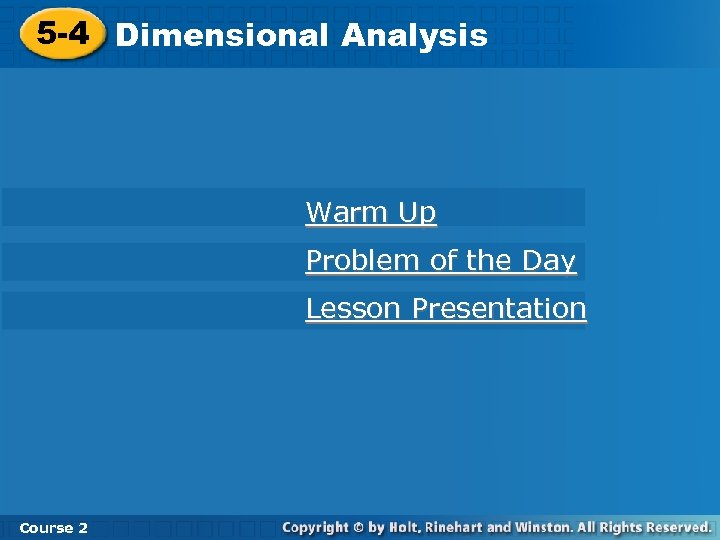5 -4 Dimensional Analysis Warm Up Problem of the Day Lesson Presentation Course 2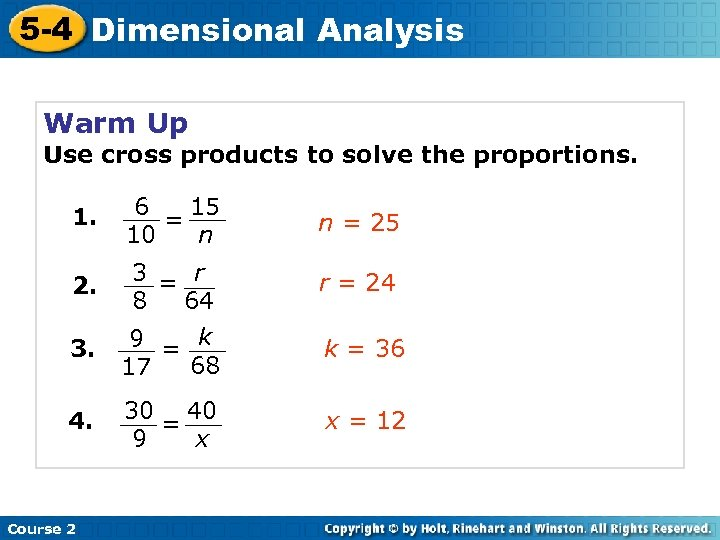5 -4 Dimensional Analysis Warm Up Use cross products to solve the proportions. 1. 2. 3. 4. Course 2 6 15 = 10 n n = 25 3 = r 8 64 9 = k 68 17 r = 24 30 40 = x 9 x = 12 k = 36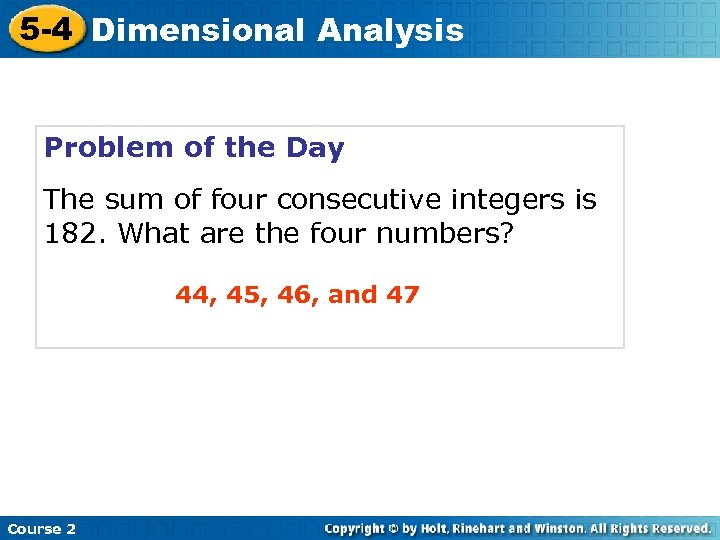5 -4 Dimensional Analysis Problem of the Day The sum of four consecutive integers is 182. What are the four numbers? 44, 45, 46, and 47 Course 2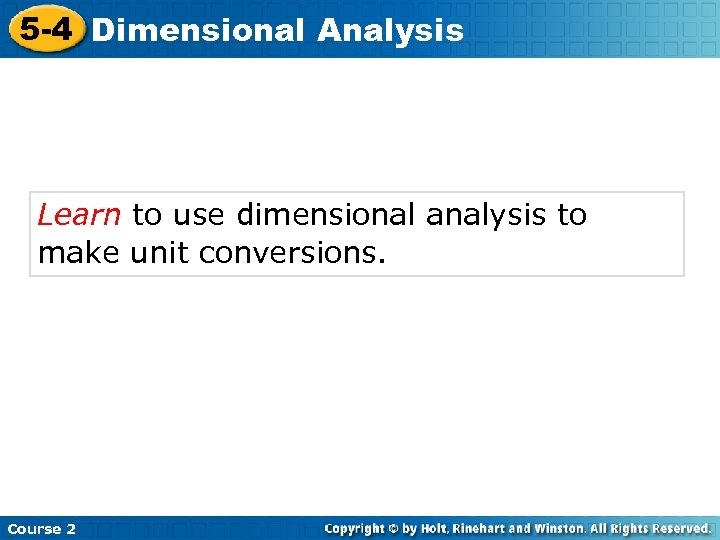5 -4 Dimensional Analysis Learn to use dimensional analysis to make unit conversions. Course 2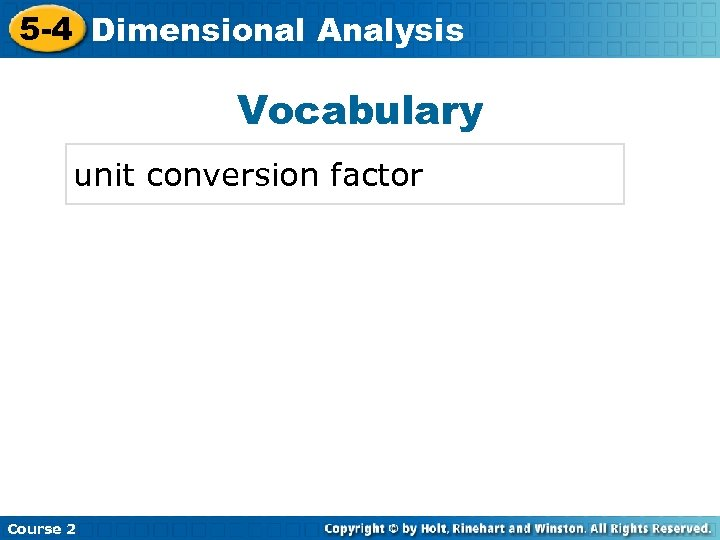5 -4 Dimensional Analysis Insert Lesson Title Here Vocabulary unit conversion factor Course 2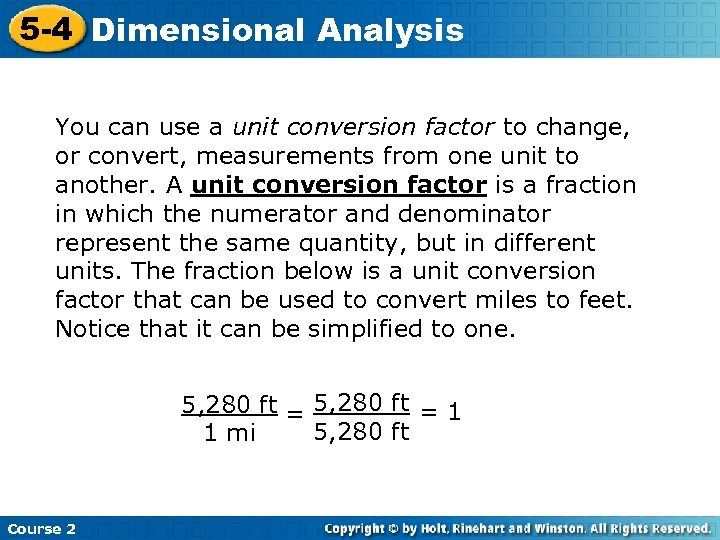5 -4 Dimensional Analysis You can use a unit conversion factor to change, or convert, measurements from one unit to another. A unit conversion factor is a fraction in which the numerator and denominator represent the same quantity, but in different units. The fraction below is a unit conversion factor that can be used to convert miles to feet. Notice that it can be simplified to one. 5, 280 ft = 1 5, 280 ft 1 mi Course 2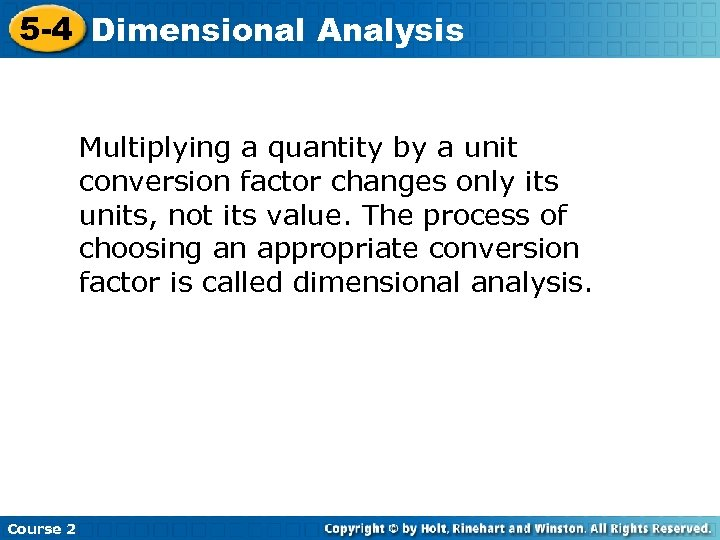5 -4 Dimensional Analysis Multiplying a quantity by a unit conversion factor changes only its units, not its value. The process of choosing an appropriate conversion factor is called dimensional analysis. Course 2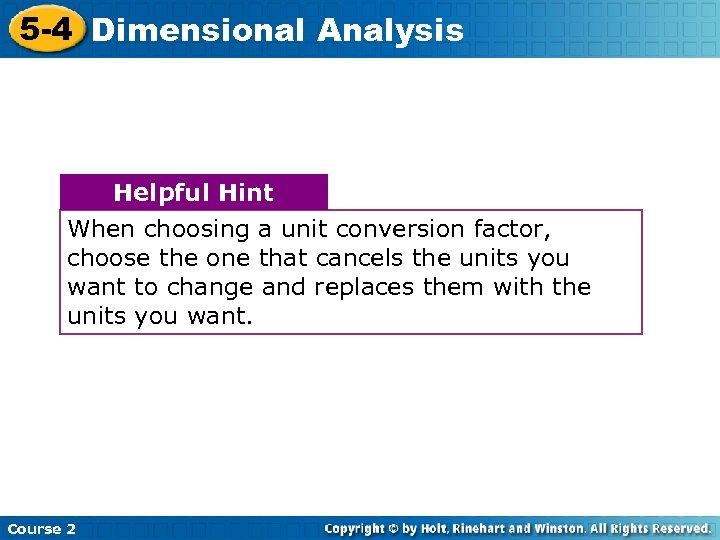5 -4 Dimensional Analysis Helpful Hint When choosing a unit conversion factor, choose the one that cancels the units you want to change and replaces them with the units you want. Course 2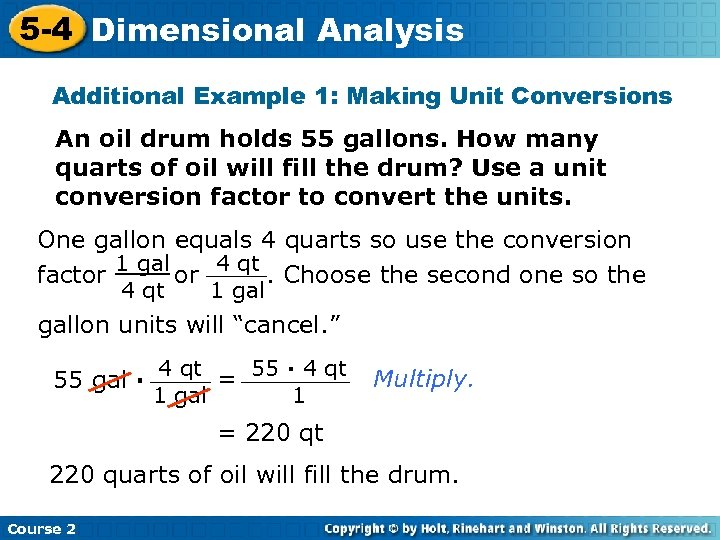5 -4 Dimensional Analysis Additional Example 1: Making Unit Conversions An oil drum holds 55 gallons. How many quarts of oil will fill the drum? Use a unit conversion factor to convert the units. One gallon equals 4 quarts so use the conversion factor 1 gal or 4 qt. Choose the second one so the 4 qt 1 gallon units will “cancel. ” 55 gal · 4 qt = 55 · 4 qt 1 gal 1 Multiply. = 220 qt 220 quarts of oil will fill the drum. Course 2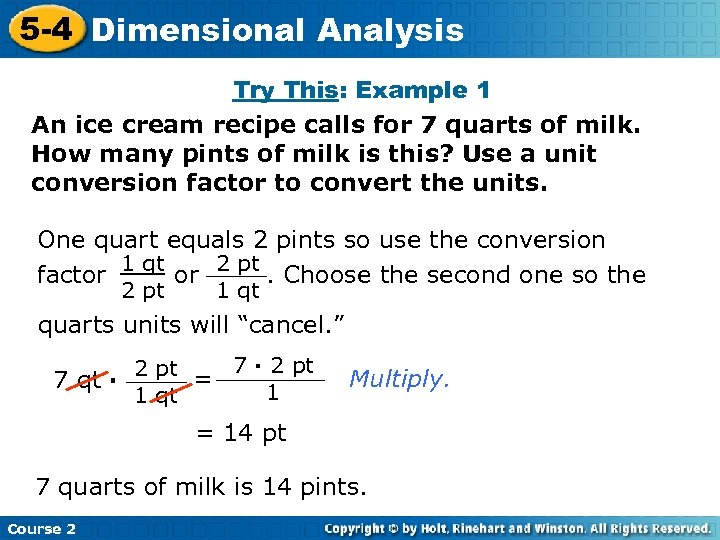5 -4 Dimensional Analysis Try This: Example 1 An ice cream recipe calls for 7 quarts of milk. How many pints of milk is this? Use a unit conversion factor to convert the units. One quart equals 2 pints so use the conversion factor 1 qt or 2 pt. Choose the second one so the 2 pt 1 qt quarts units will “cancel. ” 2 pt = 7 · 2 pt 7 qt · 1 1 qt Multiply. = 14 pt 7 quarts of milk is 14 pints. Course 2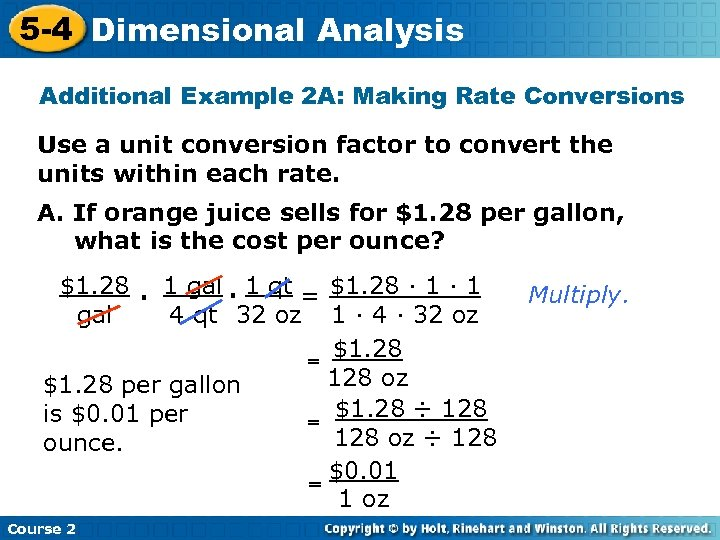5 -4 Dimensional Analysis Additional Example 2 A: Making Rate Conversions Use a unit conversion factor to convert the units within each rate. A. If orange juice sells for \$1. 28 per gallon, what is the cost per ounce? \$1. 28 · 1 gal · 1 qt = \$1. 28 · 1 gal 4 qt 32 oz 1 · 4 · 32 oz \$1. 28 = 128 oz \$1. 28 per gallon \$1. 28 ÷ 128 is \$0. 01 per = 128 oz ÷ 128 ounce. \$0. 01 = 1 oz Course 2 Multiply.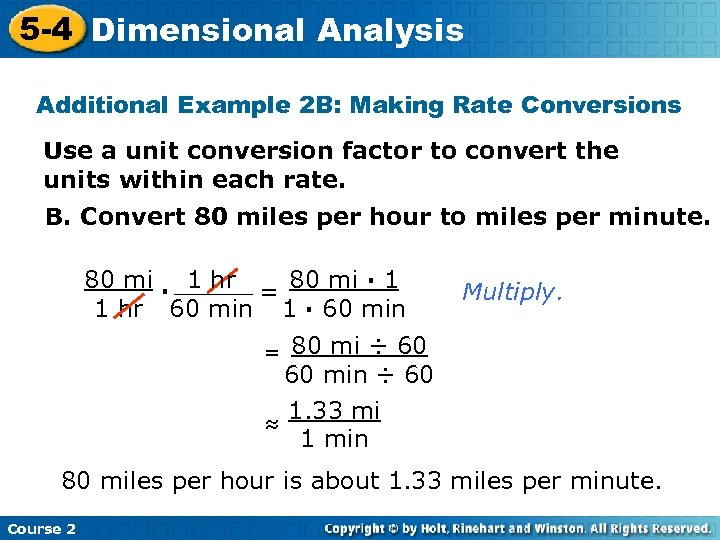5 -4 Dimensional Analysis Insert Lesson Title Here Additional Example 2 B: Making Rate Conversions Use a unit conversion factor to convert the units within each rate. B. Convert 80 miles per hour to miles per minute. 80 mi · 1 hr 80 mi · 1 = 1 hr 60 min 1 · 60 min Multiply. 80 mi ÷ 60 60 min ÷ 60 1. 33 mi ≈ 1 min = 80 miles per hour is about 1. 33 miles per minute. Course 2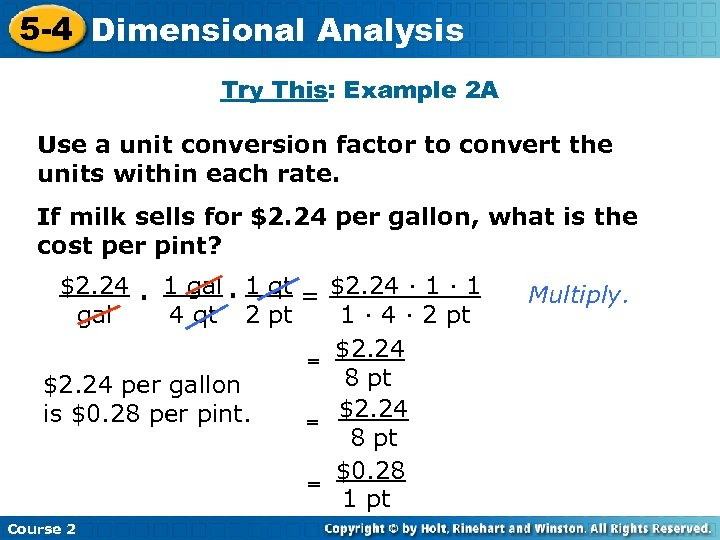5 -4 Dimensional Analysis Try This: Example 2 A Use a unit conversion factor to convert the units within each rate. If milk sells for \$2. 24 per gallon, what is the cost per pint? \$2. 24 · 1 gal · 1 qt = \$2. 24 · 1 gal 4 qt 2 pt 1 · 4 · 2 pt \$2. 24 = 8 pt \$2. 24 per gallon \$2. 24 is \$0. 28 per pint. = 8 pt \$0. 28 = 1 pt Course 2 Multiply.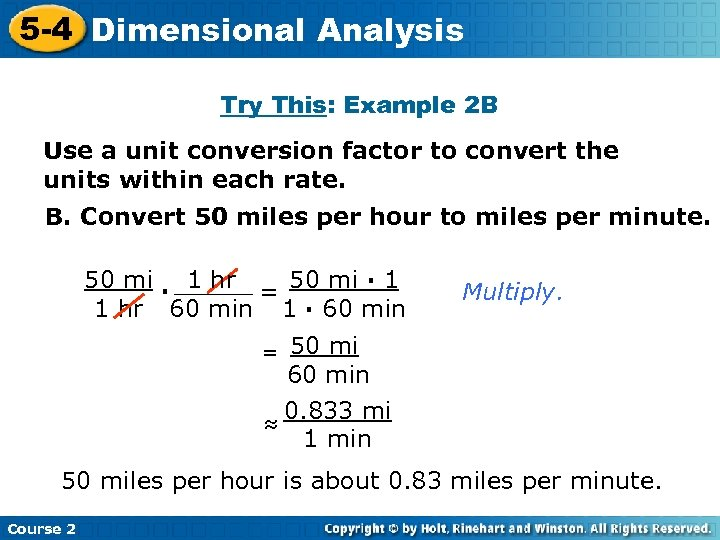5 -4 Dimensional Analysis Insert Lesson Title Here Try This: Example 2 B Use a unit conversion factor to convert the units within each rate. B. Convert 50 miles per hour to miles per minute. 50 mi · 1 hr 50 mi · 1 = 1 hr 60 min 1 · 60 min Multiply. 50 mi 60 min 0. 833 mi ≈ 1 min = 50 miles per hour is about 0. 83 miles per minute. Course 2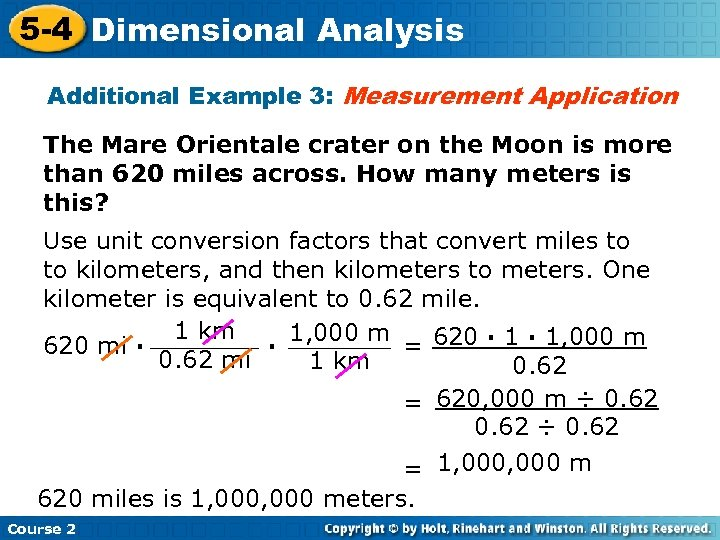5 -4 Dimensional Analysis Additional Example 3: Measurement Application The Mare Orientale crater on the Moon is more than 620 miles across. How many meters is this? Use unit conversion factors that convert miles to to kilometers, and then kilometers to meters. One kilometer is equivalent to 0. 62 mile. 1 km 1, 000 m 620 mi · · = 620 · 1, 000 m 0. 62 mi 1 km 0. 62 = 620, 000 m ÷ 0. 62 = 1, 000 m 620 miles is 1, 000 meters. Course 2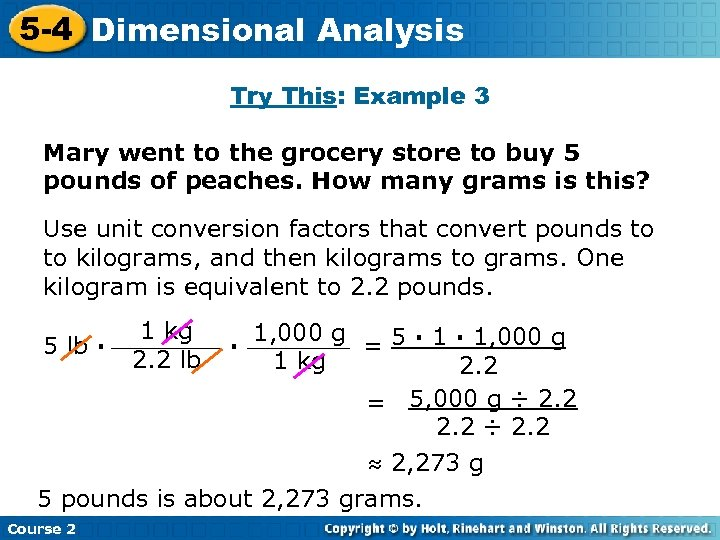5 -4 Dimensional Analysis Try This: Example 3 Mary went to the grocery store to buy 5 pounds of peaches. How many grams is this? Use unit conversion factors that convert pounds to to kilograms, and then kilograms to grams. One kilogram is equivalent to 2. 2 pounds. 1 kg 2. 2 lb 1, 000 g = 5 · 1, 000 g 1 kg 2. 2 = 5, 000 g ÷ 2. 2 ≈ 2, 273 g 5 pounds is about 2, 273 grams. 5 lb · Course 2 ·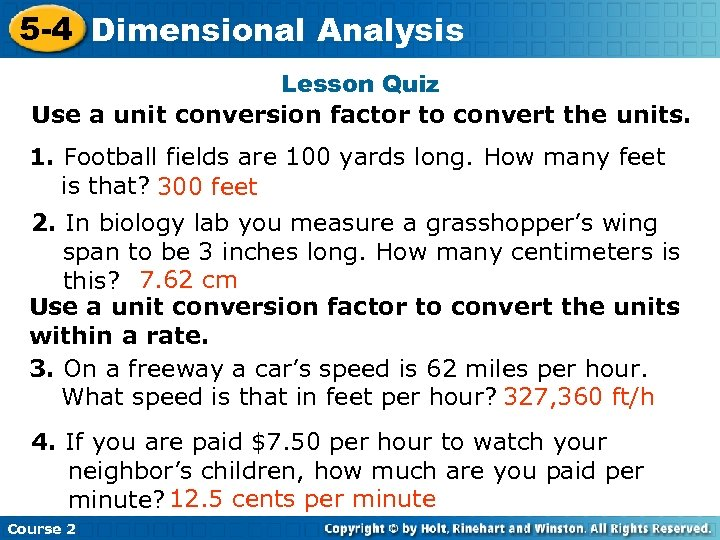5 -4 Dimensional Analysis Insert Lesson Title Here Lesson Quiz Use a unit conversion factor to convert the units. 1. Football fields are 100 yards long. How many feet is that? 300 feet 2. In biology lab you measure a grasshopper’s wing span to be 3 inches long. How many centimeters is this? 7. 62 cm Use a unit conversion factor to convert the units within a rate. 3. On a freeway a car’s speed is 62 miles per hour. What speed is that in feet per hour? 327, 360 ft/h 4. If you are paid \$7. 50 per hour to watch your neighbor’s children, how much are you paid per minute? 12. 5 cents per minute Course 2